# Subtract Numbers in Excel

There’s no SUBTRACT function in Excel. However, there are several ways to subtract numbers in Excel. Are you ready to improve your Excel skills?

1. For example, the formula below subtracts numbers in a cell. Simply use the minus sign (-). Don’t forget, always start a formula with an equal sign (=).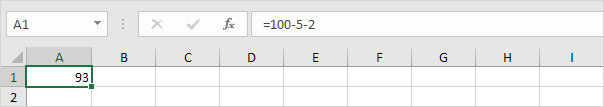2. The formula below subtracts the value in cell A2 and the value in cell A3 from the value in cell A1.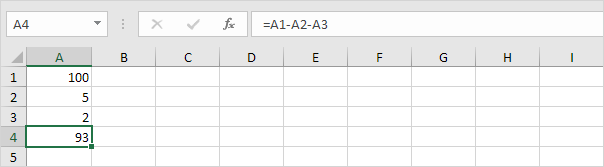3. As you can imagine, this formula can get quite long. Simply use the SUM function to shorten your formula. For example, the formula below subtracts the values in the range A2:A9 from the value in cell A1.

Worked Example:   How to calculate total from percentage in Excel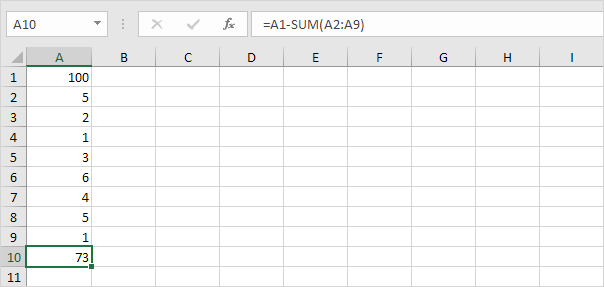Take a look at the screenshot below. To subtract the numbers in column B from the numbers in column A, execute the following steps.

4a. First, subtract the value in cell B1 from the value in cell A1.

Worked Example:   Find Percentage Change Between Numbers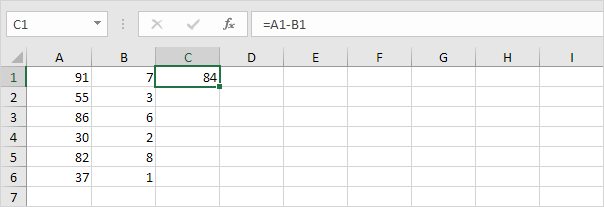4b. Next, select cell C1, click on the lower right corner of cell C1 and drag it down to cell C6.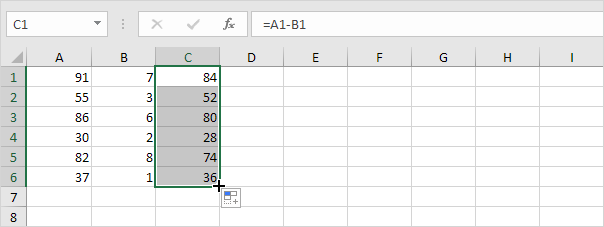Take a look at the screenshot below. To subtract a number from a range of cells, execute the following steps.

5a. First, subtract the value in cell A8 from the value in cell A1. Fix the reference to cell A8 by placing a \$ symbol in front of the column letter and row number (\$A\$8).

Worked Example:   How To Create Budget in Excel5b. Next, select cell B1, click on the lower right corner of cell B1 and drag it down to cell B6.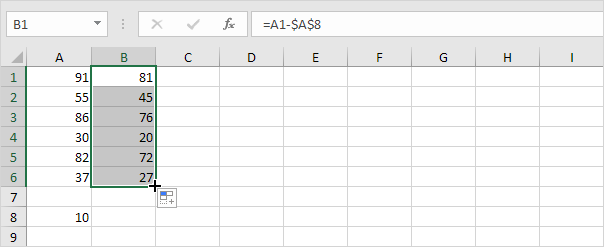Explanation: when we drag the formula down, the absolute reference (\$A\$8) stays the same, while the relative reference (A1) changes to A2, A3, A4, etc. Maybe this is one step too far for you at this stage, but it shows you one of the many other powerful features Excel has to offer.